# LeetCode周賽296，難度較低的新手練習場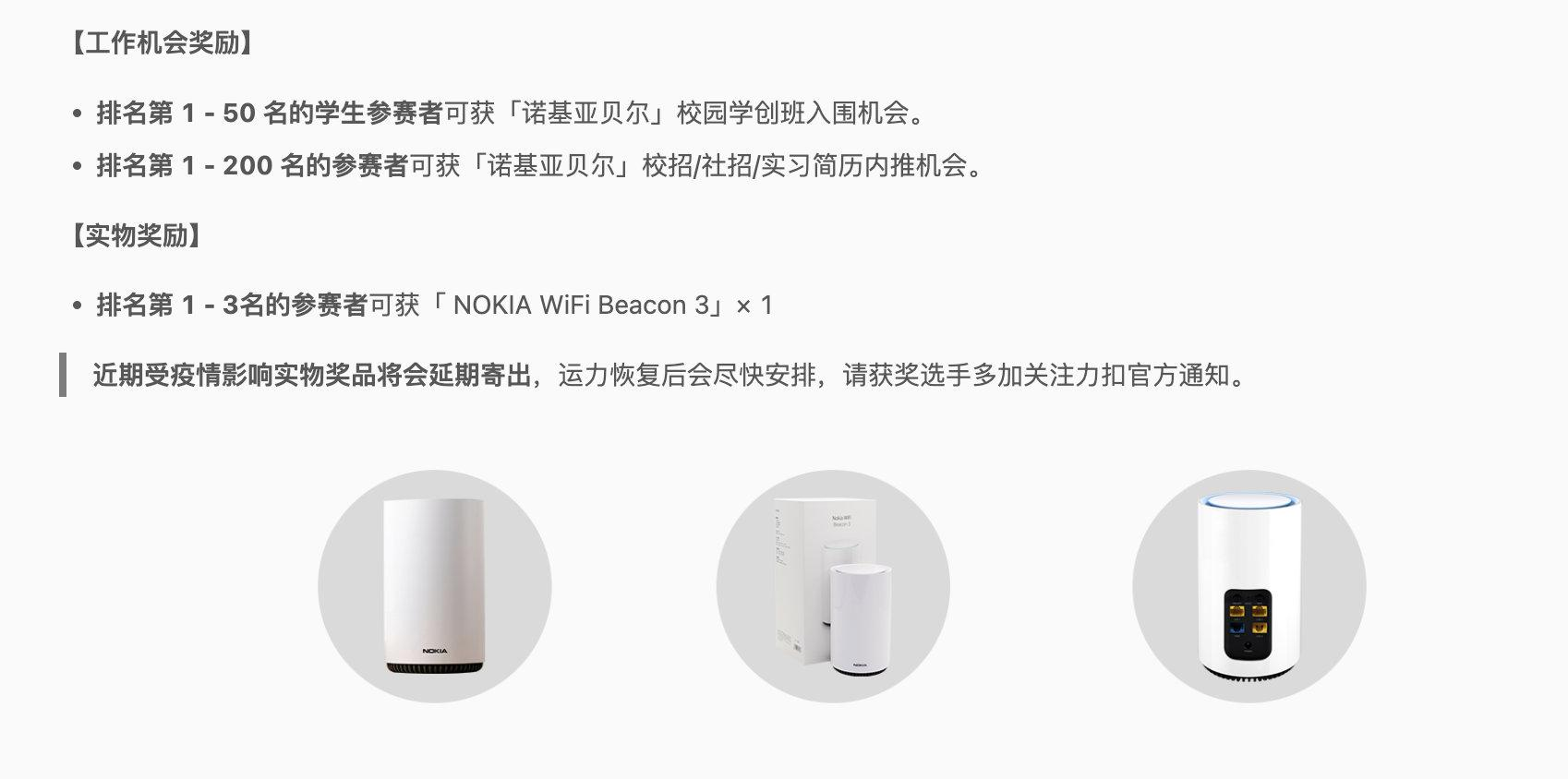## 極大極小遊戲

1. 設 n 等於 nums 的長度，如果 n == 1 ，終止 演算法過程。否則，建立 一個新的整數陣列 newNums ，新陣列長度為 n / 2 ，下標從 0 開始。
2. 對於滿足 0 <= i < n / 2 的每個 偶數 下標 i ，將 newNums[i] 賦值 為 min(nums[2 * i], nums[2 * i + 1]) 。
3. 對於滿足 0 <= i < n / 2 的每個 奇數 下標 i ，將 newNums[i] 賦值 為 max(nums[2 * i], nums[2 * i + 1]) 。
4. 用 newNums 替換 nums 。
5. 從步驟 1 開始 重複 整個過程。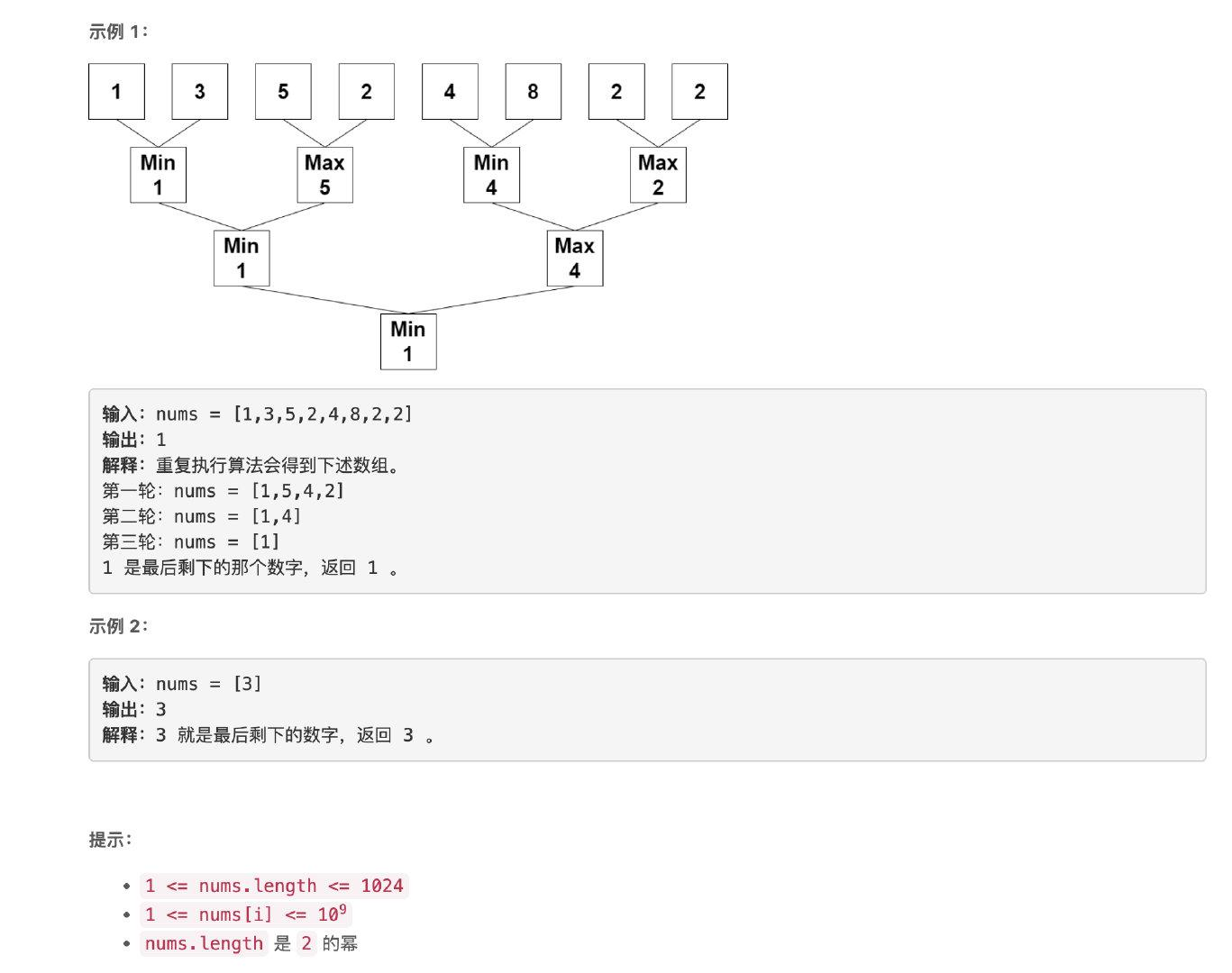### 題解

```python class Solution: def minMaxGame(self, nums: List[int]) -> int: n = len(nums)

``````    while n > 1:
news = []
for i in range(n // 2):
if i % 2 == 0:
news.append(min(nums[i*2], nums[i*2+1]))
else:
news.append(max(nums[i*2], nums[i*2+1]))
nums = news
n = len(nums)
return nums
``````

```

## 劃分陣列使最大差為 K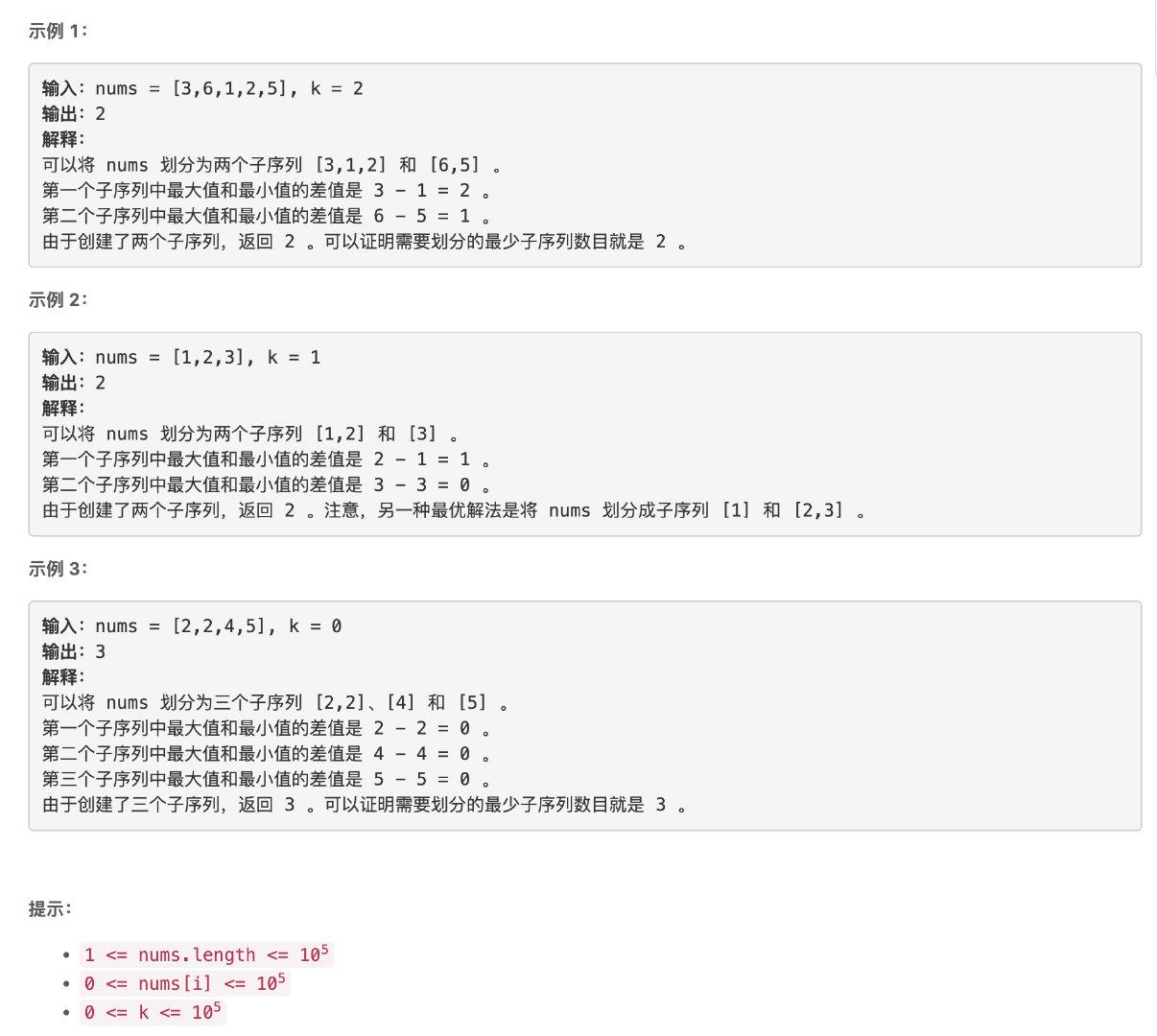### 題解

```cpp class Solution { public: int partitionArray(vector& nums, int k) { int n = nums.size();

``````    sort(nums.begin(), nums.end());

int last = nums;
int ret = 1;
for (int i = 1; i < n; i++) {
if (nums[i] > last + k) {
ret++;
last = nums[i];
}
}
return ret;
}
``````

}; ```

## 替換陣列中的元素

• operations[i] 在 nums 中存在。
• operations[i] 在 nums 中不存在。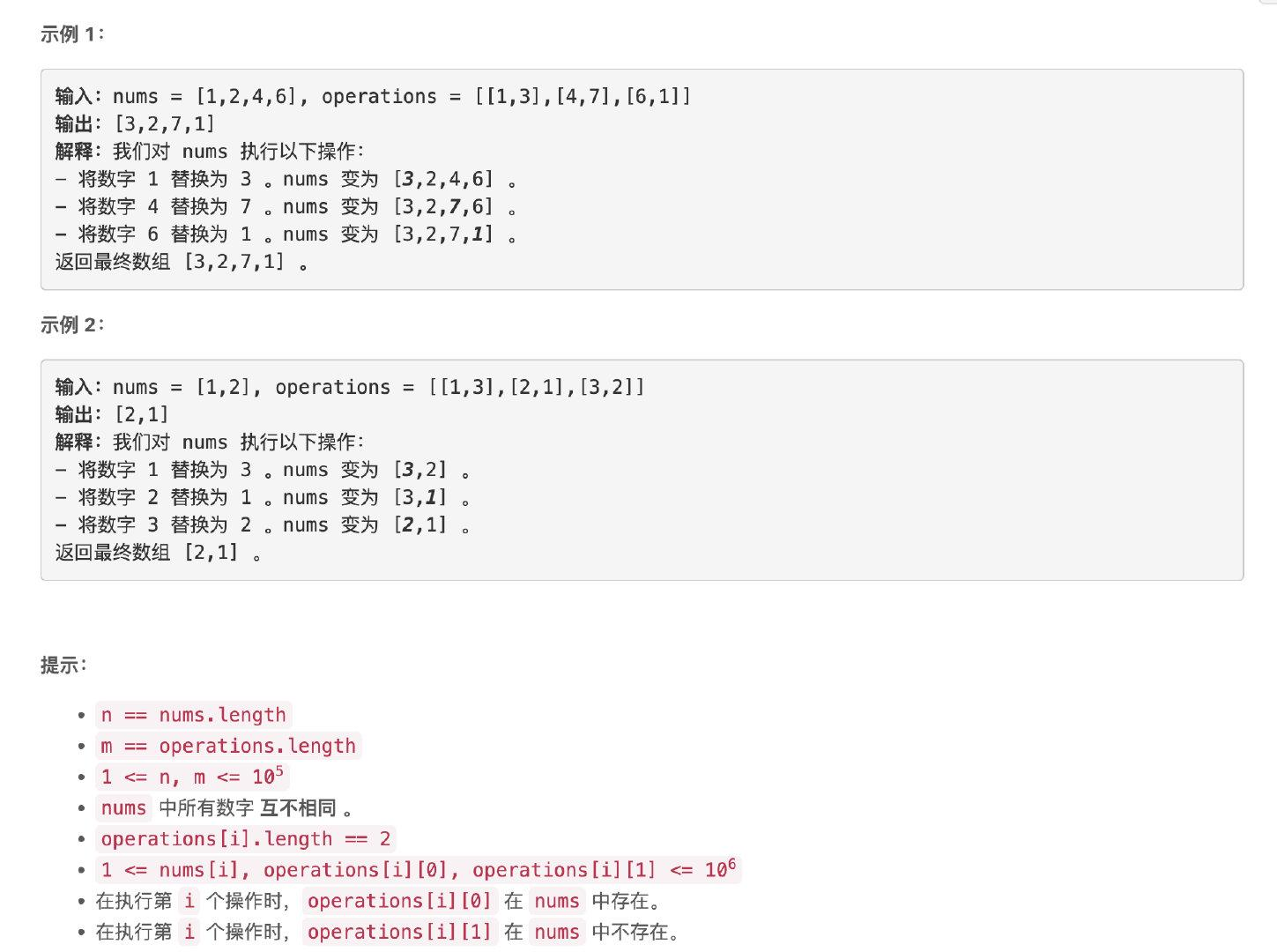### 題解

```cpp class Solution { public: vector arrayChange(vector& nums, vector>& operations) { map fwd, bkd; for (auto &op : operations) { int u = op, v = op; if (bkd.count(u) == 0) { fwd[u] = v; bkd[v] = u; }else { int pu = bkd[u]; fwd[pu] = v; bkd[v] = pu; bkd.erase(u); } }

``````    int n = nums.size();
for (int i = 0; i < n; i++) {
int u = nums[i];
if (fwd.count(u)) nums[i] = fwd[u];
}
return nums;
}
``````

}; ```

## 設計一個文字編輯器

• 新增：在游標所在處新增文字。
• 刪除：在游標所在處刪除文字（模擬鍵盤的刪除鍵）。
• 移動：將游標往左或者往右移動。

• TextEditor() 用空文字初始化物件。
• void addText(string text) 將 text 新增到游標所在位置。新增完後游標在 text 的右邊。
• int deleteText(int k) 刪除游標左邊 k 個字元。返回實際刪除的字元數目。
• string cursorLeft(int k) 將游標向左移動 k 次。返回移動後游標左邊 min(10, len) 個字元，其中 len 是游標左邊的字元數目。
• string cursorRight(int k) 將游標向右移動 k 次。返回移動後游標左邊 min(10, len) 個字元，其中 len 是游標左邊的字元數目。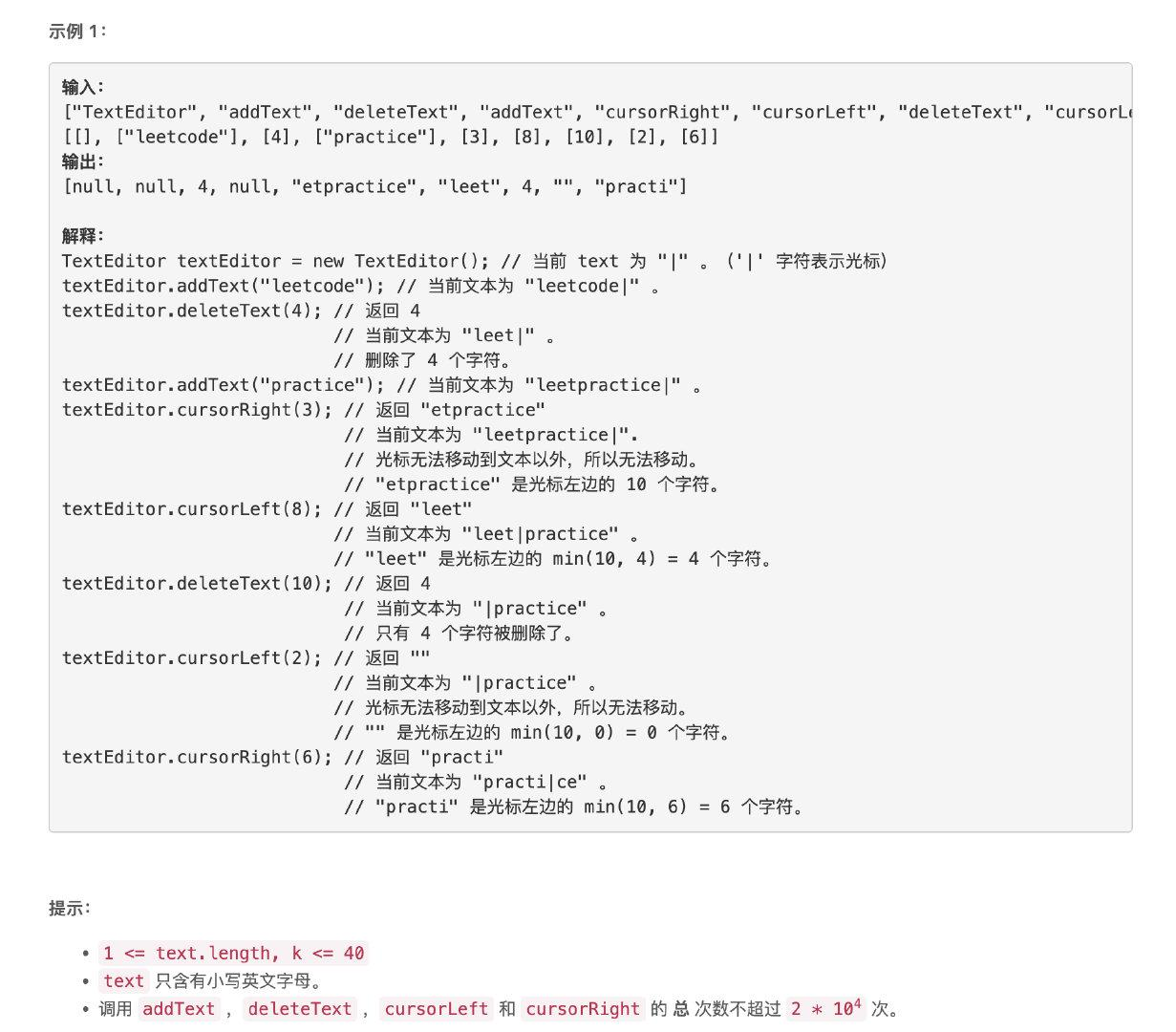### 題解

```cpp class TextEditor { public:

``````string cl, cr;
int p;

TextEditor() {
p = 0;
}

for (auto c : text) {
cl.push_back(c);
}
}

int deleteText(int k) {
int ret = 0;
// 刪除，等價於彈出游標左側的末尾元素
for (int i = 0; i < k; i++) {
if (cl.empty()) break;
ret++;
cl.pop_back();
}
return ret;
}

string cursorLeft(int k) {
// 向左移動游標，等價於刪除游標左側的內容，插入到右側
for (int i = 0; i < k; i++) {
if (cl.empty()) break;
char c = cl.back();
cl.pop_back();
cr.push_back(c);
}

string ret = "";
for (int i = max((int) cl.size()-10, 0); i < cl.size(); i++) ret.push_back(cl[i]);
return ret;
}

string cursorRight(int k) {
// 向右移動游標，等價於彈出游標右側的內容，插入到左側
for (int i = 0; i < k; i++) {
if (cr.empty()) break;
char c = cr.back();
cr.pop_back();
cl.push_back(c);
}

string ret = "";
for (int i = max((int) cl.size()-10, 0); i < cl.size(); i++) ret.push_back(cl[i]);
return ret;
}
``````

};

/ * Your TextEditor object will be instantiated and called as such: * TextEditor obj = new TextEditor(); * obj->addText(text); * int param_2 = obj->deleteText(k); * string param_3 = obj->cursorLeft(k); * string param_4 = obj->cursorRight(k); / ```